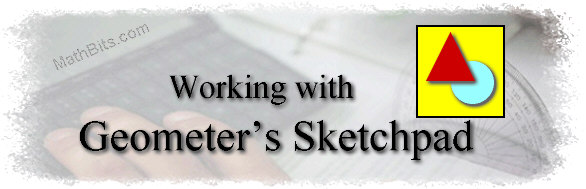Angles and Parallel Lines

Step-by-step directions for constructing and investigating angles in relation to parallel lines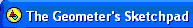Version 4.+
Investigating angles with parallel and non-parallel lines.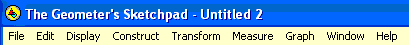1. Set Preferences:  Under Edit, choose "Preferences", the "Units" Tab (indicate cm and hundredths), "Text" Tab (if necessary, turn off the automatic labeling = un-check box under "Show Label Automatically" in front of "for all new points") and "Color" Tab (pick any color choices).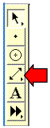2. Draw a line:  The Segment Tool (Straightedge) extends to the right to include a Line Tool.  The Line Tool will automatically create a line through two points. Label the points A and B.  A right click on each point will drop the box shown at the right.  Choose "Label Point". right click drop box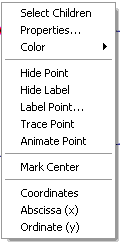3.  Draw another line:  Draw another line that does not intersect the first line (AB) on the screen but does not appear parallel to the first line.  Label this line's points C and D. 4.  Draw transversal:  Draw a third line, a transversal, which intersects both lines, passing between A and B and between C and D.   Label this line's points E and F.

5. Points of intersection:  Establish the two points of intersection where the transversal crosses the first two lines.  Choose the Select Tool,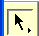, click to highlight line AB and line EF,  choose Construct menu, option Intersection.  Label the point.  Repeat for CD and EF.   (You can also establish the intersection point by using the point tool.)

6. Measure angles:  Choose the Select Tool, click to highlight the three points of any angle whose vertex is one of the two points of intersection with the transversal.  From the Measure menu, choose Angle.  Repeat for all eight angles. Arrange the angle measures appropriately on the screen.   At this point you should see a diagram similar to: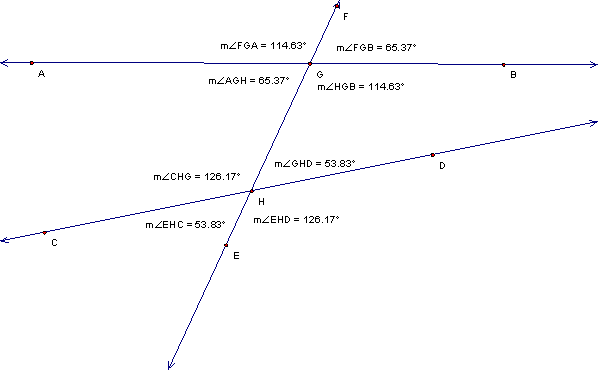7.  Stop and Observe:  The lines in this drawing are not parallel.  What observations can be made regarding the alternate interior angles, the corresponding angles, the interior angles on the same side of the transversal, the alternate exterior angles, the vertical angles, and the angles forming linear pairs?

8.  Draw Parallel Lines:  Choose the Select tool, highlight line CD.  Under Edit, choose Cut.  Highlight the intersection point E and line AB.  Choose the Construct menu, Parallel Line.

9.  Measure Angles:  Measure the eight angles again.

10.  Stop and Observe:  The lines in this drawing are parallel.  What observations can be made regarding the alternate interior angles, the corresponding angles, the interior angles on the same side of the transversal, the alternate exterior angles, the vertical angles, and the angles forming linear pairs?

11.  Complete the observation sheet.